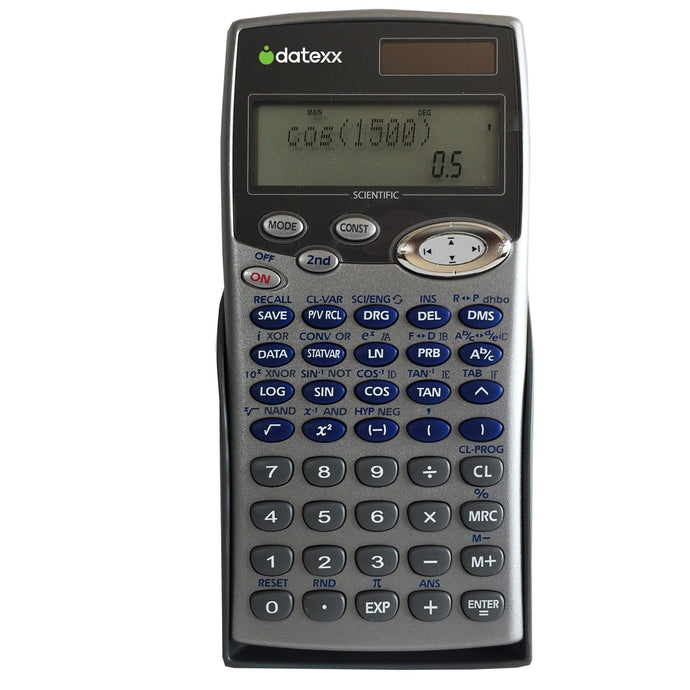DS-834

# Solar powered 455-Function, 2 Line, Fraction, Equation Scientific Calculator

Regular price \$26.00 Sale

Datexx DS-834

Full Comparison to TI-34/36

 DS-834 Key Features * Total 20 Scientific Constants and 170 Metric Conversions * Statistical and Regression (Capability of Production CP,CPK and Normal Distribution) * Variable Linear and Quadratic Equation solving * Logical Calculations (Calculates/Converts in DEC, HEX, OCT, BIN) * Polar/Rectangular coordinate transformation * Fraction and Complex number calculations * Up to 24 Calculating Digits * 455 Functions (429 functions excluding memory) * Max. 21 Pending Values * Max. 30 Pending Operations * 8 steps Store/Recall

This unit is ideal for Mathematic Calculations, Trigonometry, Statistics, Science,
Computer math, and Algebraic 1&2 functions.

• Easy to read clear function keys combined with the assistance of a step-by-step
instruction manual to make learning scientific calculations almost painless!
• In addition to the convenience of a two line display, the unit features a
conventional battery power source backed up by a build-in ''fail-safe''
solar panel. No lost data!

### Key Features

• 148 Bytes program, 13 Parenthese level, 30 Max. number of pending operation, Integration, Deferentiation
• 20 Scientific Constant,170 metric conversion,Formula, ENG SYM,Recover
• Compare to TI-34 & TI-36

### Specifications

• Dimensions: 3*6*0.5(inches) / 7.62*15.24*1.27 (cm)
• Battery: 1 x LR1130